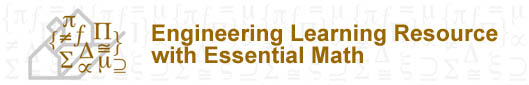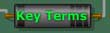Learning Resource Topics Overview 1 Charge & Coulomb’s Law 2 Electric Current 3 Voltage 4 Resistance 5 Ohm’s Law 6 Power & Energy 7 Elements of Electric Circuits 8 Kirchhoff’s Law 9 Series Circuits 10 Parallel Circuits 11 Analysis of Combination Circuits 12 Capacitance 13 Inductance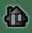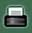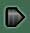# Topic 1

Charge is the quantity of electricity responsible for electric phenomena. It is one of the fundamental physical quantities in electric circuit analysis.

Coulomb’s law is the fundamental law of the electric force (F) between two stationary, charged particles. It states that the force of electrostatic attraction or repulsion is directly proportional to the product of the two charges and inversely proportional to the square of the distance between them. Coulomb’s law may also introduce another concept that is the electric field intensity (E).

# Topic 2

Current is the time rate of change of electric charge passing through a predetermined area.

Current density is the measure of the amount of current flowing through a unit area normal to the direction of current flow.

A direct current (DC) is a current of constant magnitude in time.

A sinusoidal current (AC) is that varies sinusoidaly with time.

Frequency is the number of times the direction of current changes (and changes back) per second.

# Topic 3

Voltage across an element is the electrical pressure, measured in volts (V), i.e., the electromotive force (EMF), which causes current to flow through an electric circuit.

# Topic 4

Resistance is the most common component in electric circuits. It is used mainly to control current and voltage within the circuit. It is measured in ohms.

A passive element, however, is defined as an element that cannot supply an average power that is greater than zero over an infinite time interval.

Conductance is the ability of an element to conduct electric current; it is measured in mhos or siemens (S).

# Topic 5

Ohm’s Law deals with the relationship between voltage and current in an ideal conductor. This relationship states that the potential difference v across a conductor of resistance R is proportional to the current i through it.

# Topic 6

Power is the time rate of expending or absorbing energy, measured in watts (W).

Passive sign convention is satisfied when the current enters through the positive terminal of an element and p = +vi . If the current enters through the negative terminal, then p = -vi.

Energy is the capacity to do work. It is measured in joules (J).

# Topic 7

An electric circuit or electric network is an interconnection of electrical elements linked together in a closed path so that electric current may flow continuously.

A reference point is an arbitrarily chosen point to which all other points in the circuit are compared.

An open circuit is a circuit element whose resistance approaches infinity R = ) and accordingly no current can flow in the circuit.

A short circuit is a circuit that has very little or no resistance to limit the flow of current.

A grounded circuit is a path of current other than the intended that is established to ground.

A source is a voltage or current generator capable of supplying electrical energy to a circuit.

Independent source is a source in which the voltage is independent of the current, or the current is independent of the voltage. It is termed as active elements.

Dependent source is a source for which either the source voltage or current depends upon a voltage or current elsewhere in the circuit. It is termed as active elements.

An active element is an element that is capable of furnishing an average power greater than zero to some external devices, where the average is taken over an infinite time interval.

# Topic 8

Kirchhoff’s current law (KCL) states that at any node in a circuit, the current arriving is equal to the current leaving.

Kirchhoff’s voltage law (KVL)
states that the algebraic sum of the voltage drops in any closed path in a circuit and the electromotive forces in that path are equal to zero.

# Topic 9

The elements of a series circuit are connected end to end; the same current flows through its parts one after another.

A voltage divider is a circuit that works on the principle that the sum of the voltage drops across a series circuit must equal the applied voltage. Voltage dividers may be constructed to produce any voltage desired.

# Topic 10

The elements of a parallel circuit are connected so that each component has the same voltage across its terminals; the current flow is divided among its parts.

# Topic 11

A combination circuit is a circuit that contains a combination of both series and parallel elements.

Circuit analysis is the process of solving a circuit in order to determine voltages across, or the current through the elements of the circuit.

# Topic 12

The capacitor is a passive component. It is defined as the ability to store an electric charge.

# Topic 13

An inductor is defined as the ability of a coil (consisting of multiple turns of wire wound in a helical geometry around a core) to store magnetic energy, induce a voltage in itself, and oppose changes in current flowing through it.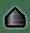Content and Pedagogy© 2004, University of Ottawa, Faculty of Engineering and Faculty of Education
Design and Production © 2004, University of Ottawa, Centre for e-Learning